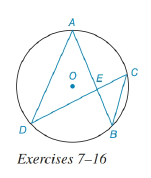Chapter 6.3, Problem 12E### Elementary Geometry for College St...

6th Edition
Daniel C. Alexander + 1 other
ISBN: 9781285195698

#### Solutions

Chapter
Section### Elementary Geometry for College St...

6th Edition
Daniel C. Alexander + 1 other
ISBN: 9781285195698
Textbook Problem
1 views

# In the figure for Exercises 7 to 16, O is the center of the circle. See Theorem 6.3.5.Given: A D = 10 , B C = 4 , A E = 7 Find: EC.To determine

To find:

The value of EC.

Explanation

The diagrammatic representation is given below,

Theorem:

1. If two chords intersect within a circle, then the product of the lengths of the segments (parts) of one chord is equal to the product of the lengths of the segments of the other chord. Now compare the triangles ΔAED,ΔCEB

2. If two inscribed angles intercept the same arc, then these angles congruent. By using the above theorem mADE=mCBE

Vertical angles are congruent to each other. The angles that are opposite to each other are vertical angles.

By suing the vertical angle theorem to get the following,

mAED=mCEB

Since by Angle rule we conclude that

m

### Still sussing out bartleby?

Check out a sample textbook solution.

See a sample solution

#### The Solution to Your Study Problems

Bartleby provides explanations to thousands of textbook problems written by our experts, many with advanced degrees!

Get Started

#### Let f be the function defined by f(t)=2t2t1. Find f(2), f(a), f(x + 1), and f(x 1).

Applied Calculus for the Managerial, Life, and Social Sciences: A Brief Approach

#### Convert the expressions in Exercises 8596 radical form. y7/4

Finite Mathematics and Applied Calculus (MindTap Course List)

#### True or False: If f(x) 0 for x c and f(x) 0 for x c, then c is a point of inflection.

Study Guide for Stewart's Single Variable Calculus: Early Transcendentals, 8th

#### True or False: , for –π ≤ t ≤ π, is smooth.

Study Guide for Stewart's Multivariable Calculus, 8th